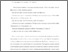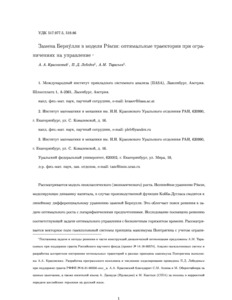Bernoulli substitution in the Ramsey model: Optimal trajectories under control constraints

, Lebedev, P.D., & Tarasyev, A.M. (2017). Bernoulli substitution in the Ramsey model: Optimal trajectories under control constraints. Computational Mathematics and Mathematical Physics 57 (5) 770-783. 10.1134/S0965542517050050.Preview
Text (In Russian)
manuscript_Krasovskii_et_al.pdf - Accepted Version

Abstract

We consider a neoclassical (economic) growth model. A nonlinear Ramsey equation, modeling capital dynamics, in the case of Cobb-Douglas production function is reduced to the linear differential equation via a Bernoulli substitution. This considerably facilitates the search for a solution to the optimal growth problem with logarithmic preferences. The study deals with solving the corresponding infinite horizon optimal control problem. We consider a vector field of the Hamiltonian system in the Pontryagin maximum principle, taking into account control constraints. We prove the existence of two alternative steady states, depending on the constraints. A proposed algorithm for constructing growth trajectories combines methods of open-loop control and closed-loop regulatory control. For some levels of constraints and initial conditions, a closed-form solution is obtained. We also demonstrate the impact of technological change on the economic equilibrium dynamics. Results are supported by computer calculations.

Item Type: Article Published in Russian in Zhurnal Vychislitel’noi Matematiki i Matematicheskoi Fiziki, 2017, Vol. 57, No. 5, pp. 768–782. mathematical modeling, optimal growth problem, Pontryagin’s maximum principle, steady states Ecosystems Services and Management (ESM) Luke Kirwan 12 Jun 2017 08:24 27 Aug 2021 17:29 http://pure.iiasa.ac.at/14661View Item